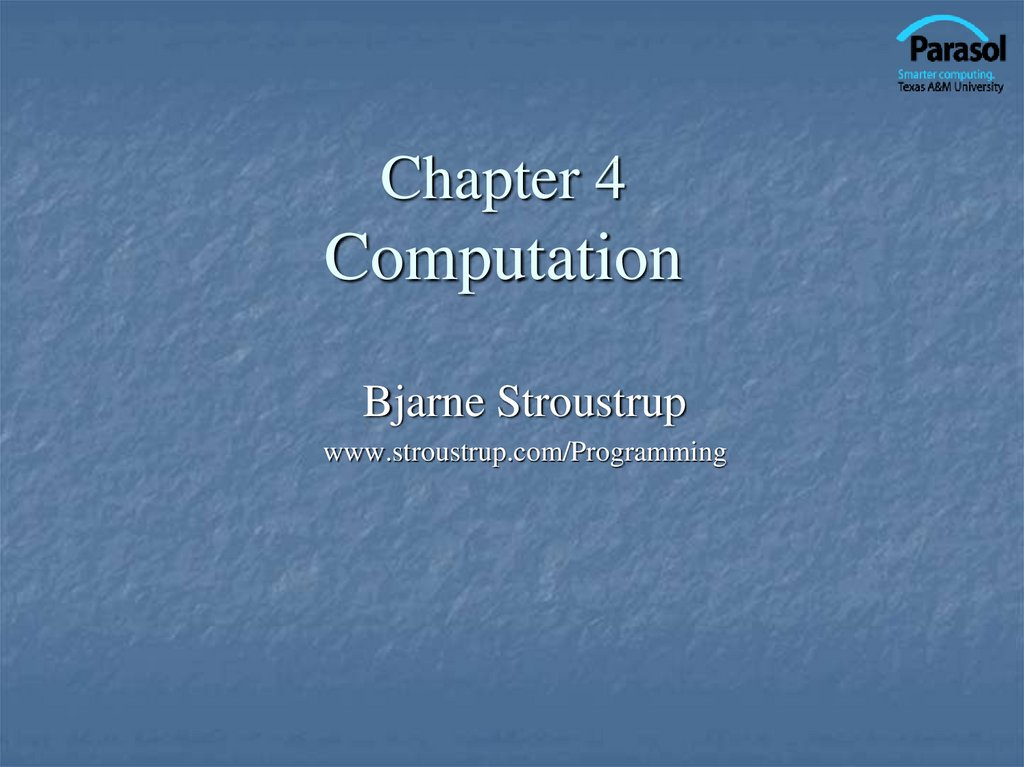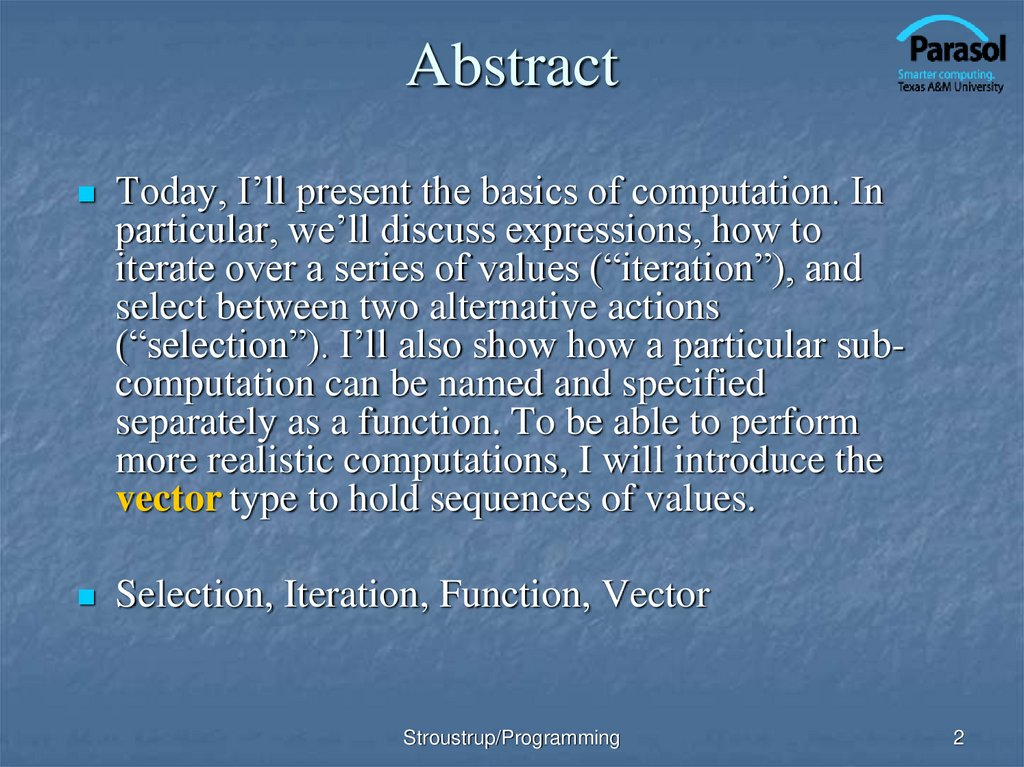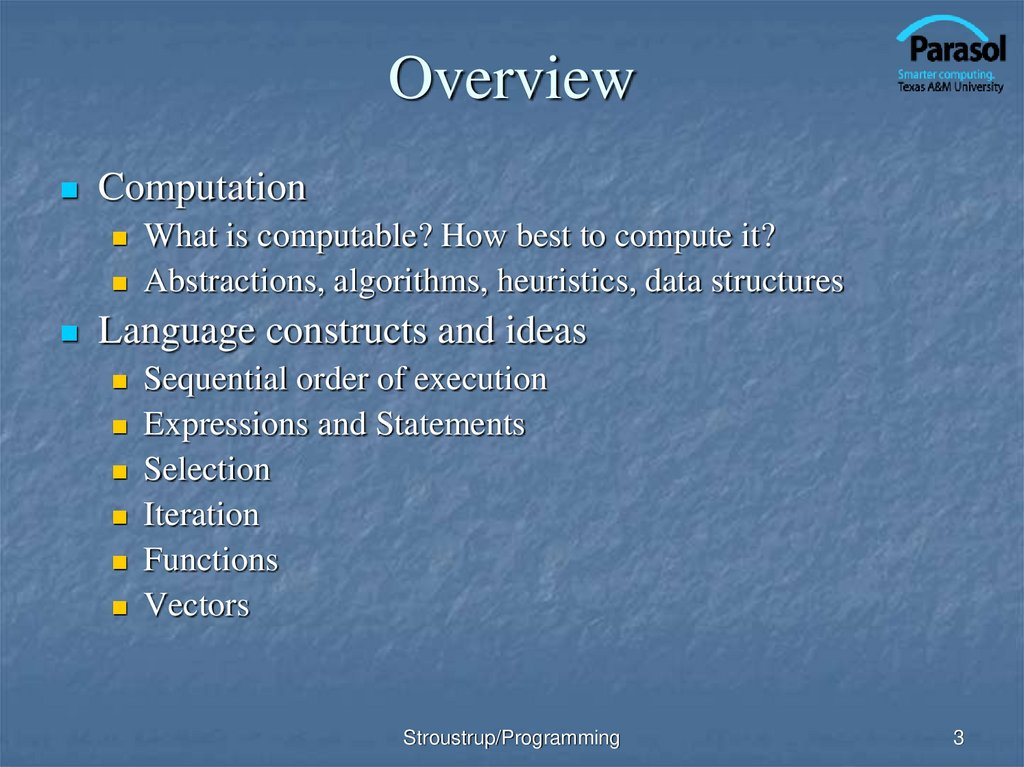# Chapter 4 Computation

## 1. Chapter 4 Computation

Bjarne Stroustrup
www.stroustrup.com/Programming

## 2. Abstract

Today, I’ll present the basics of computation. In
particular, we’ll discuss expressions, how to
iterate over a series of values (“iteration”), and
select between two alternative actions
(“selection”). I’ll also show how a particular subcomputation can be named and specified
separately as a function. To be able to perform
more realistic computations, I will introduce the
vector type to hold sequences of values.
Selection, Iteration, Function, Vector
Stroustrup/Programming
2

## 3. Overview

Computation
What is computable? How best to compute it?
Abstractions, algorithms, heuristics, data structures
Language constructs and ideas
Sequential order of execution
Expressions and Statements
Selection
Iteration
Functions
Vectors
Stroustrup/Programming
3

## 4. You already know most of this

Note:
You know how to do arithmetic
d = a+b*c
You know how to select
“if this is true, do that; otherwise do something else ”
You know how to “iterate”
You know how to do functions
“do this until you are finished”
“do that 100 times”
“hey Joe, calculate this for me and send me the answer”
What I will show you today is mostly just vocabulary and
syntax for what you already know
Stroustrup/Programming
4

## 5. Computation

Code, often messy,
(input) data
often a lot of code
(output) data
data
Input: from keyboard, files, other input devices, other programs, other
parts of a program
Computation – what our program will do with the input to produce the
output.
Output: to screen, files, other output devices, other programs, other parts
of a program
Stroustrup/Programming
5

## 6. Computation

Our job is to express computations
One tool is called Divide and Conquer
Provide a higher-level concept that hides detail
Organization of data is often the key to good code
to break up big computations into many little ones
Another tool is Abstraction
Correctly
Simply
Efficiently
Input/output formats
Protocols
Data structures
Note the emphasis on structure and organization
You don’t get good code just by writing a lot of statements
Stroustrup/Programming
6

## 7. Language features

Each programming language feature exists to express
a fundamental idea
For example
* : multiplication
if (expression) statement else statement ; selection
while (expression) statement ;
iteration
f(x);
function/operation

We combine language features to create programs
Stroustrup/Programming
7

## 8. Expressions

// compute area:
int length = 20;
// the simplest expression: a literal (here, 20)
// (here used to initialize a variable)
int width = 40;
int area = length*width;
int average = (length+width)/2;
// a multiplication
The usual rules of precedence apply:
a*b+c/d means (a*b)+(c/d) and not a*(b+c)/d.
If in doubt, parenthesize. If complicated, parenthesize.
Don’t write “absurdly complicated” expressions:
a*b+c/d*(e-f/g)/h+7
// too complicated
Choose meaningful names.
Stroustrup/Programming
8

## 9. Expressions

Expressions are made out of operators and operands
Operators specify what is to be done
Operands specify the data for the operators to work with
Boolean type: bool
(true and false)
Equality operators: = = (equal), != (not equal)
Logical operators: && (and), || (or), ! (not)
Relational operators: < (less than), > (greater than), <=, >=
Character type: char (e.g., 'a', '7', and '@')
Integer types: short, int, long
arithmetic operators: +, -, *, /, % (remainder)
Floating-point types: e.g., float, double (e.g., 12.45 and 1.234e3)
arithmetic operators: +, -, *, /
Stroustrup/Programming
9

## 10. Concise Operators

For many binary operators, there are (roughly) equivalent
more concise operators
For example
a += c
means
a = a+c
a *= scale
means
a = a*scale
++a
means
a += 1
or
a = a+1
“Concise operators” are generally better to use
(clearer, express an idea more directly)
Stroustrup/Programming
10

## 11. Statements

A statement is
For example
an expression followed by a semicolon, or
a declaration, or
a “control statement” that determines the flow of control
a = b;
double d2 = 2.5;
if (x == 2) y = 4;
while (cin >> number) numbers.push_back(number);
int average = (length+width)/2;
return x;
You may not understand all of these just now, but you will …
Stroustrup/Programming
11

## 12. Selection

Sometimes we must select between alternatives
For example, suppose we want to identify the larger of two
values. We can do this with an if statement
if (a<b)
max = b;
else
max = a;
// Note: No semicolon here
// Note: No semicolon here
The syntax is
if (condition)
statement-1
else
statement-2
// if the condition is true, do statement-1
// if not, do statement-2
Stroustrup/Programming
12

## 13. Iteration (while loop)

The world’s first “real program” running on a stored-program
computer (David Wheeler, Cambridge, May 6, 1949)
// calculate and print a table of squares 0-99:
int main()
{
int i = 0;
while (i<100) {
cout << i << '\t' << square(i) << '\n';
++i ;
// increment i
}
}
// (No, it wasn’t actually written in C++ .)
Stroustrup/Programming
13

## 14. Iteration (while loop)

What it takes
A loop variable (control variable);
Initialize the control variable;
A termination criterion;
Increment the control variable;
Something to do for each iteration;
here: i
here: int i = 0
here: if i<100 is false, terminate
here: ++i
here: cout << …
int i = 0;
while (i<100) {
cout << i << '\t' << square(i) << '\n';
++i ;
// increment i
}
Stroustrup/Programming
14

## 15. Iteration (for loop)

Another iteration form: the for loop
You can collect all the control information in one
place, at the top, where it’s easy to see
for (int i = 0; i<100; ++i) {
cout << i << '\t' << square(i) << '\n';
}
That is,
for (initialize; condition ; increment )
controlled statement
Note: what is square(i)?
Stroustrup/Programming
15

## 16. Functions

But what was square(i)?
A call of the function square()
int square(int x)
{
return x*x;
}
We define a function when we want to separate a
computation because it
is logically separate
makes the program text clearer (by naming the computation)
is useful in more than one place in our program
eases testing, distribution of labor, and maintenance
Stroustrup/Programming
16

## 17. Control Flow

int main()
{
int square(int x)
i=0;
{
while (i<100)
{
return x * x;
}
square(i)
i<100
}
}
i==100
Stroustrup/Programming
17

## 18. Functions

Our function
int square(int x)
{
return x*x;
}
is an example of
Return_type function_name ( Parameter list )
// (type name, etc.)
{
// use each parameter in code
return some_value;
// of Return_type
}
Stroustrup/Programming
18

## 19. Another Example

Earlier we looked at code to find the larger of two values.
Here is a function that compares the two values and returns
the larger value.
int max(int a, int b) // this function takes 2 parameters
{
if (a<b)
return b;
else
return a;
}
int x = max(7, 9);
int y = max(19, -27);
int z = max(20, 20);
// x becomes 9
// y becomes 19
// z becomes 20
Stroustrup/Programming
19

## 20. Data for Iteration - Vector

To do just about anything of interest, we need a collection of
data to work on. We can store this data in a vector. For example:
// read some temperatures into a vector:
int main()
{
vector<double> temps;
// declare a vector of type double to store
// temperatures – like 62.4
double temp;
// a variable for a single temperature value
while (cin>>temp)
// cin reads a value and stores it in temp
temps.push_back(temp);
// store the value of temp in the vector
// … do something …
}
// cin>>temp will return true until we reach the end of file or encounter
// something that isn’t a double: like the word “end”
Stroustrup/Programming
20

## 21. Vector

Vector is the most useful standard library data type
a vector<T> holds an sequence of values of type T
Think of a vector this way
A vector named v contains 5 elements: {1, 4, 2, 3, 5}:
size()
v:
5
v’s elements:
v
v
v
v
v
1
4
2
3
5
Stroustrup/Programming
21

## 22. Vectors

vector<int> v;
v:
// start off empty
0
v.push_back(1); // add an element with the value 1
v:
1
1
v.push_back(4); // add an element with the value 4 at end (“the back”)
v:
2
1
4
v.push_back(3); // add an element with the value 3 at end (“the back”)
v
v:
3
v
1
Stroustrup/Programming
v
4
3
22

## 23. Vectors

Once you get your data into a vector you can easily manipulate it:
// compute mean (average) and median temperatures:
int main()
{
vector<double> temps;
// temperatures in Fahrenheit, e.g. 64.6
double temp;
while (cin>>temp) temps.push_back(temp); // read and put into vector
double sum = 0;
for (int i = 0; i< temps.size(); ++i) sum += temps[i];
// sums temperatures
cout << "Mean temperature: " << sum/temps.size() << endl;
sort(temps.begin(),temps.end());
cout << "Median temperature: " << temps[temps.size()/2] << endl;
}
Stroustrup/Programming
23

## 24. Combining Language Features

You can write many new programs by combining
language features, built-in types, and user-defined
types in new and interesting ways.
So far, we have
Variables and literals of types bool, char, int, double
vector, push_back(), [ ] (subscripting)
!=, ==, =, +, -, +=, <, &&, ||, !
max( ), sort( ), cin>>, cout<<
if, for, while
You can write a lot of different programs with these
language features! Let’s try to use them in a slightly
different way…
Stroustrup/Programming
24

## 25. Example – Word List

// “boilerplate” left out
vector<string> words;
string s;
while (cin>>s && s != "quit")
words.push_back(s);
sort(words.begin(), words.end());
// && means AND
// sort the words we read
for (int i=0; i<words.size(); ++i)
cout<<words[i]<< "\n";
/*
read a bunch of strings into a vector of strings, sort
them into lexicographical order (alphabetical order),
and print the strings from the vector to see what we have.
*/
Stroustrup/Programming
25

## 26. Word list – Eliminate Duplicates

// Note that duplicate words were printed multiple times. For
// example “the the the”. That’s tedious, let’s eliminate duplicates:
vector<string> words;
string s;
while (cin>>s && s!= "quit") words.push_back(s);
sort(words.begin(), words.end());
for (int i=1; i<words.size(); ++i)
if(words[i-1]==words[i])
“get rid of words[i]” // (pseudocode)
for (int i=0; i<words.size(); ++i) cout<<words[i]<< "\n";
// there are many ways to “get rid of words[i]”; many of them are messy
// (that’s typical). Our job as programmers is to choose a simple clean
// solution – given constraints – time, run-time, memory.
Stroustrup/Programming
26

## 27. Example (cont.) Eliminate Words!

// Eliminate the duplicate words by copying only unique words:
vector<string> words;
string s;
while (cin>>s && s!= "quit") words.push_back(s);
sort(words.begin(), words.end());
vector<string>w2;
if (0<words.size()) {
// Note style { }
w2.push_back(words);
for (int i=1; i<words.size(); ++i)
if(words[i-1]!=words[i])
w2.push_back(words[i]);
}
cout<< "found " << words.size()-w2.size() << " duplicates\n";
for (int i=0; i<w2.size(); ++i) cout << w2[i] << "\n";
Stroustrup/Programming
27

## 28. Algorithm

We just used a simple algorithm
An algorithm is (from Google search)
“a logical arithmetical or computational procedure that, if correctly
applied, ensures the solution of a problem.” – Harper Collins
“a set of rules for solving a problem in a finite number of steps, as for
finding the greatest common divisor.” – Random House
“a detailed sequence of actions to perform or accomplish some task.
Named after an Iranian mathematician, Al-Khawarizmi. Technically, an
algorithm must reach a result after a finite number of steps, …The term is
also used loosely for any sequence of actions (which may or may not
terminate).” – Webster’s
We eliminated the duplicates by first sorting the vector
(so that duplicates are adjacent), and then copying only
strings that differ from their predecessor into another
vector.
Stroustrup/Programming
28

## 29. Ideal

Basic language features and libraries should be
usable in essentially arbitrary combinations.
We are not too far from that ideal.
If a combination of features and types make sense,
it will probably work.
The compiler helps by rejecting some absurdities.
Stroustrup/Programming
29

## 30. The next lecture

How to deal with errors
Stroustrup/Programming
30